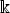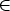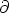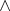### DIPS 02/2002 [tex source, PostScript, PDF file, dvi]

Title: On n-ary generalizations of the Lie algebra sl2()

Author: Arthemy V. Kiselev

Polynomials ajN[x], 1 <j<N, of degree deg aj = N are shown to be closed w.r.t. action of the N-ary bracket [a1, ..., aN] = W(a1,...,aN ), where W denotes the Wronskian determinant. This bracket is proved to induce the homotopical N-Lie algebra structure on the polynomialsN[x] of degree N, so that an extended Jacobi identity (with 2N-1 summands) holds; the case N = 2 is the Lie bracket in sl2() satisfying the Jacobi identity.

The property of the Wronskian determinants to compose the homotopical N-Lie algebras is proved to hold for arbitrary analytic functions aj[[x]], thus extending the former result. The identity [[i1...ik+1,j1...jl+1]]RN = 0 is discussed, whereis a derivation and [[.,.]]RN is the Richardson-Nijenhuis bracket.

The notion of the Wronskian determinant is generalized to the case of n independent variables: (x1,...,xn)n, so that the resulting concept preserves the homotopical N-Lie Jacobi identity.

12 pages, LaTeX-2e (AmS-LaTeX).

To compile source files (.tex) one needs the title page style files titlatex.tex.